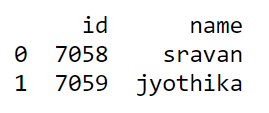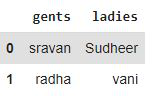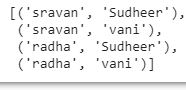# Pandas – All combinations of two columns

• Last Updated : 15 Mar, 2021

In this article, we will see how to get the combination of two columns of a DataFrame. First, let’s create a sample DataFrame.

Code: An example code to create a data frame using dictionary.

## Python3

 `# importing pandas module for the  ``# data frame``import` `pandas as pd`` ` `# creating data frame for student details ``# using dictionary``data ``=` `pd.DataFrame({``'id'``: [``7058``, ``7059``, ], ``                     ``'name'``: [``'sravan'``, ``'jyothika'``]})`` ` `print``(data)`

Output:To combine two columns in a data frame using itertools module. It provides various functions that work on iterators to produce complex iterators. To get all combinations of columns we will be using itertools.product module. This function computes the cartesian product of input iterables. To compute the product of an iterable with itself, we use the optional repeat keyword argument to specify the number of repetitions. The output of this function is tuples in sorted order.

Syntax: itertools.product(iterables, repeat=1)

Code:

## Python3

 `# import pandas as pd``import` `pandas as pd`` ` `# creating data frame``df ``=` `pd.DataFrame(data``=``[[``'sravan'``, ``'Sudheer'``], ``                        ``[``'radha'``, ``'vani'``], ],``                  ``columns``=``[``'gents'``, ``'ladies'``])`` ` `print``(df)`

Output:Code:

## Python3

 `# importing product``from` `itertools ``import` `product`` ` `# apply product method``print``(``list``(product(df[``'gents'``], df[``'ladies'``])))`

Output:My Personal Notes arrow_drop_up